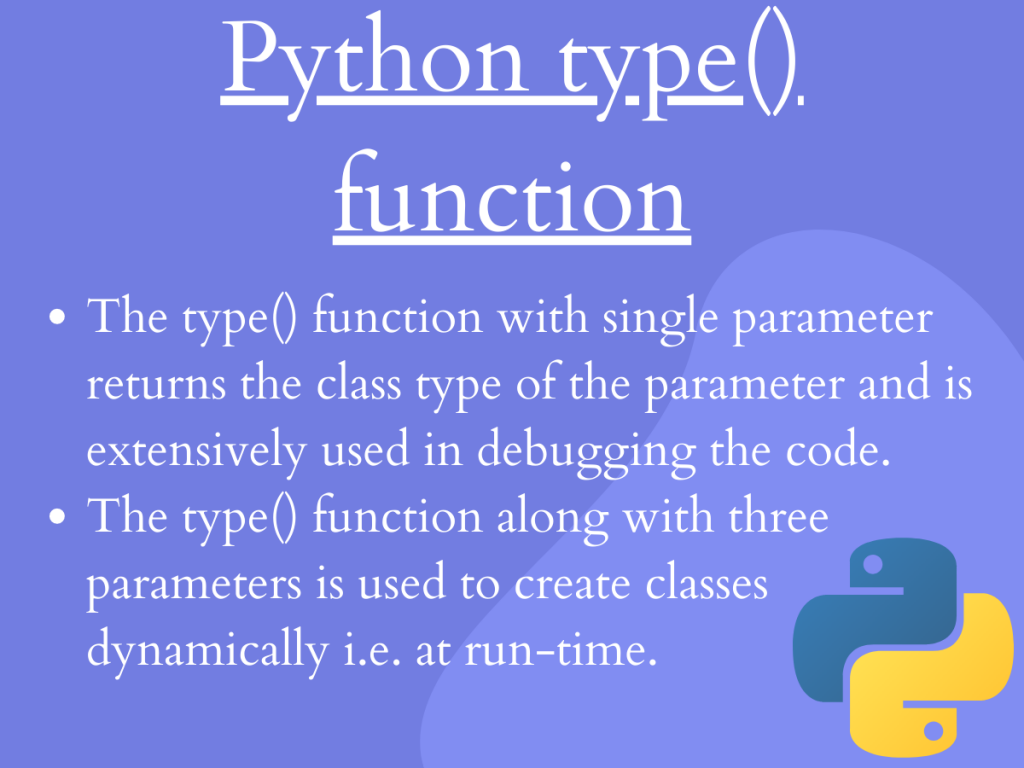# Variants of Python type() functionHey, folks! In this article, we will having a look at one of the important built-in function of Python in terms of debuggingPython type() function.

## Getting started with Python type() function

Python type() function serves the purpose of debugging through-out the program. The type() function can be used to debug the data type of various classes and data variables throughout the code.

The type() function can be represented in two variants–

• The type() function with one argument
• The type() function with three arguments

In the upcoming section, we will understand the functioning of both the variants of type() function in Python.

## 1. Python type() with One Argument

When a single argument is passed to the type() function, it returns the data type of the given class/object, respectively.

Syntax:

```type(object)
```
• It accepts only a single argument.
• The type() function with single parameter returns the class type of the object passed to it.

Example:

```dict_map = {"Python":'A',"Java":'B',"Kotlin":'C',"Ruby":'D'}
print("The variable dict_map is of type:",type(dict_map))

list_inp = [10,20,30,40]
print("The variable list_inp is of type:",type(list_inp))

str_inp = "Python with JournalDev"
print("The variable str_inp is of type:",type(str_inp))

tup_inp = ('Bajaj', 'Tata','Royce')
print("The variable tup_inp is of type:",type(tup_inp))

```

In the above example, we have created data objects of different data structures such as dict, list, etc. Further, we have passed it to the type() function to debug the type of the objects.

Output:

```The variable dict_map is of type: <class 'dict'>
The variable list_inp is of type: <class 'list'>
The variable str_inp is of type: <class 'str'>
The variable tup_inp is of type: <class 'tuple'>
```

## 2. Python type() with Three Arguments

When three parameters are passed to the `type() function`, it creates and returns a new type of object as output to the function.

Syntax:

```type(name, bases, dict)
```

The three parameters are as follows–

• `name`: It is a string which basically represents the name of the class.
• `bases`: It is a tuple that specifies the base classes of the main class.
• `dict`: It is a ‘dictionary‘ that is used to create body of the class specified.

Thus, the type() function with the above three parameters are used to create classes dynamically at runtime.

Example:

```var1 = type('ob', (object,), dict(A='Python', B='Cpp'))

print(type(var1))
print(vars(var1))

class apply:
A = 'Python'
B = 'Cpp'

var2 = type('oc', (apply,), dict(A = 'Python', B = 'Kotlin'))
print(type(var2))
print(vars(var2))
```

In the above example, we have created classes at dynamic runtime one with a single object class and the other class with the ‘apply’ base class to it. The` vars() function` represents the __dict__ argument of a class/module.

Output:

```<class 'type'>
{'A': 'Python', 'B': 'Cpp', '__module__': '__main__', '__dict__': <attribute '__dict__' of 'ob' objects>, '__weakref__': <attribute '__weakref__' of 'ob' objects>, '__doc__': None}
<class 'type'>
{'A': 'Python', 'B': 'Kotlin', '__module__': '__main__', '__doc__': None}
```

## Summary

• The type() function with single parameter returns the class type of the parameter and is extensively used in debugging the code.
• The type() function along with three parameters is used to create classes dynamically i.e. at run-time.

## Conclusion

Thus, in this article, we have understood the working of Python type() under different parameters, respectively.

## References

• Python type() function — JournalDev